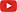## Example for discussion [Power / Sample Size]

Hi mittyri,

» Let me play the devil’s advocate.

Love it.

» Again, a man in Harmani suite as your Boss says it MUST be registered and you have only current product to test. So you are interested to register the drug (to show the bioeqivalence).
» What would be your recommendation for sample size? How would you estimate it?

Must be registered” is a bit thick! Try…

library(PowerTOST) expsampleN.TOST(CV=0.2, theta0=1.15, prior.type="both",                 prior.parm=list(m=20, design="2x2"),                 details=FALSE)

… and tell Hugo Boss that with 304 subjects there will still be a 20% risk of failure. If he wants a risk of 10% >107 subjects would be required. Since re-formulation seems not to be an option (what I strongly would recommend; see below why) you will befired.

Seriously: First I would check a CI of the CV observed in the pilot. I would use an α of 0.2.

signif(100*CVCL(CV=0.2, df=20-2, side="2-sided", alpha=0.2), 4) lower CL upper CL    16.59    25.91

OK, this is sufficiently close to the literature (18–26%). If we want to play it safe, we can use the upper CL with α 0.05 (27.94%). ”Must be registered” is not an argument which will convince the IEC and the authority assessing the protocol. You have to take the risk of getting fired and explain to Hugo Boss that a power of >90% will lead to trouble. Hence, with GMR 1.15 “carved in stone”:

sampleN.TOST(CV=CV.upper, theta0=1.15, targetpower=0.9) +++++++++++ Equivalence test - TOST +++++++++++             Sample size estimation ----------------------------------------------- Study design:  2x2 crossover log-transformed data (multiplicative model) alpha = 0.05, target power = 0.9 BE margins = 0.8 ... 1.25 True ratio = 1.15,  CV = 0.2794244 Sample size (total)  n     power 188   0.901956

Next I would fire up power analyses:

pa.ABE(CV=signif(CV.upper, 4), theta0=1.15,        targetpower=0.9, minpower=0.80) pa.ABE(CV=signif(CV.upper, 4), theta0=1.15,        targetpower=0.9, minpower=0.85)

If we accept a minimum power of 80% the GMR could rise to 1.165 and with 85% to 1.158. That’s not nice. It must be clear even to the guy in the Armani suit that performing the study though in a high sample size will be extremely risky due to the bad GMR. I’m not sure whether the protocol with such a large expected deviation will be welcomed. I know of only one case where an innovator had to change one of the excipients upon the FDA’s demand. A couple of pilots with candidate formulations performed – wasn’t possible to come up with anything better than 0.85. OK, was a blockbuster and at least they tried hard.

Dif-tor heh smusma 🖖
Helmut SchützThe quality of responses received is directly proportional to the quality of the question asked. 🚮
Science QuotesIng. Helmut Schütz